Sale!##### Mushrooms I
Currency conversion from USD to: [convert number=270.00 from="usd" to="zar"] ZAR | [convert number=270.00 from="usd" to="gbp"] GBP | [convert number=270.00 from="usd" to="eur"] EUR     Medium: Oil on Board Size: 17cm by 17cm
\$ 270.00 \$ 135.00
Sale!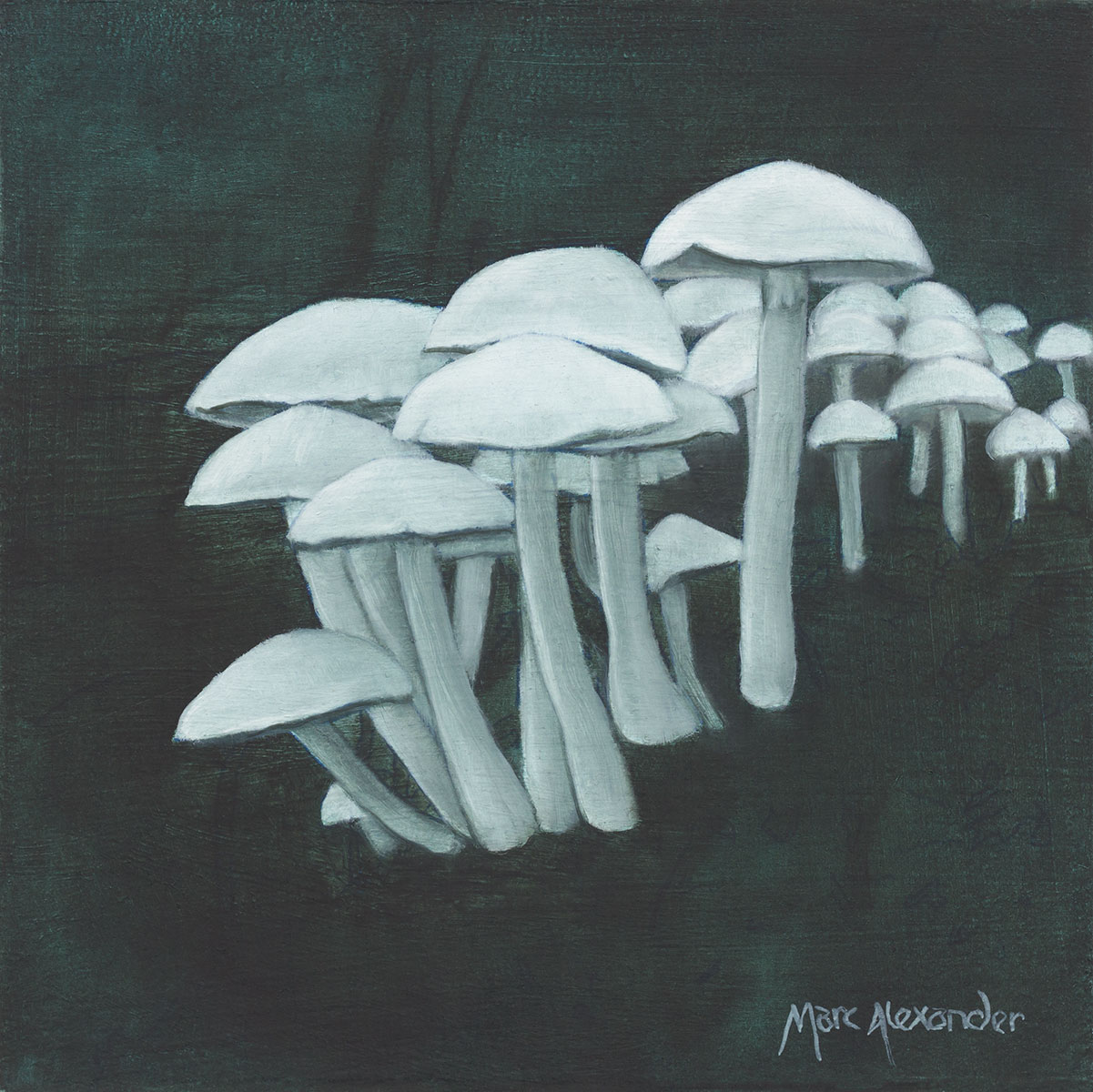##### Mushrooms II
Currency conversion from USD to: [convert number=270.00 from="usd" to="zar"] ZAR | [convert number=270.00 from="usd" to="gbp"] GBP | [convert number=270.00 from="usd" to="eur"] EUR     Medium: Oil on Board Size: 17cm by 17cm
\$ 270.00 \$ 135.00
Sale!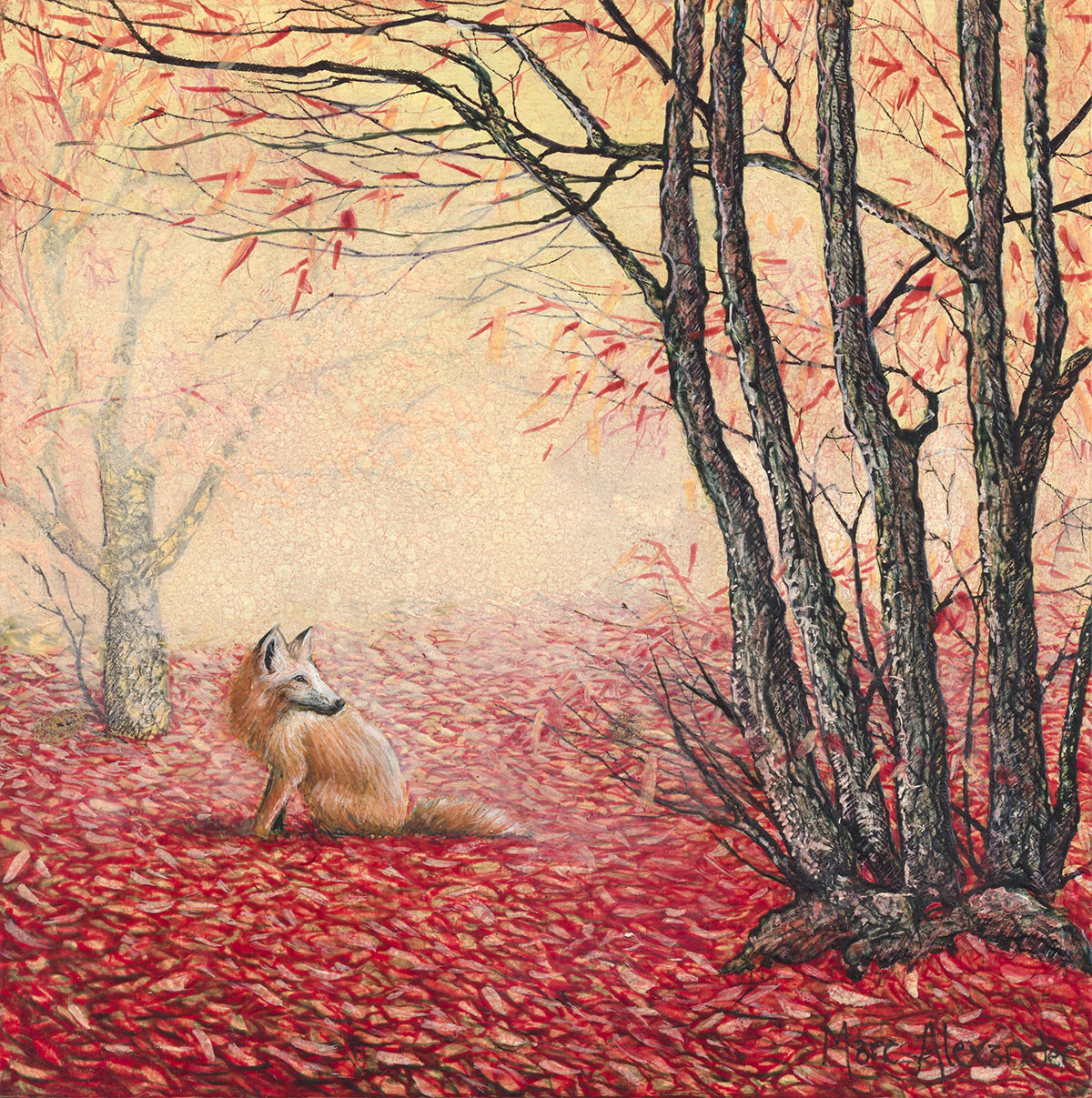##### The Red Carpet
Currency conversion from USD to: [convert number=270.00 from="usd" to="zar"] ZAR | [convert number=270.00 from="usd" to="gbp"] GBP | [convert number=270.00 from="usd" to="eur"] EUR     Medium: Oil on Board Size: 17cm by 17cm
\$ 270.00 \$ 135.00
Sale!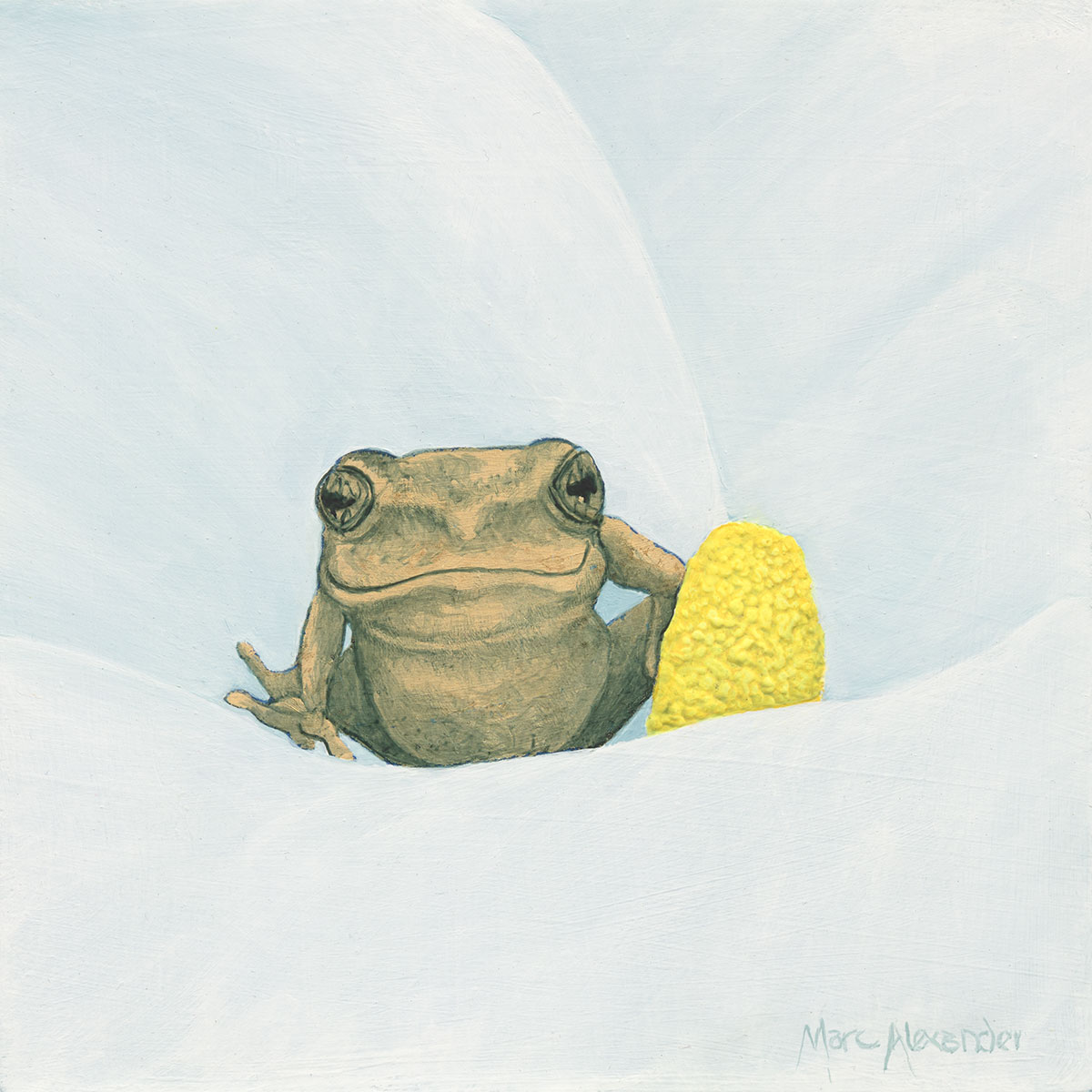##### Reed Frog I
Currency conversion from USD to: [convert number=270.00 from="usd" to="zar"] ZAR | [convert number=270.00 from="usd" to="gbp"] GBP | [convert number=270.00 from="usd" to="eur"] EUR     Medium: Oil and Gold Leaf on Board Size: 17cm by 17cm SOLD
\$ 270.00 \$ 135.00
Sale!##### Reed Frog II
Currency conversion from USD to: [convert number=270.00 from="usd" to="zar"] ZAR | [convert number=270.00 from="usd" to="gbp"] GBP | [convert number=270.00 from="usd" to="eur"] EUR     Medium: Oil and Gold Leaf on Board Size: 17cm by 17cm SOLD
\$ 270.00 \$ 135.00
Sale!##### Starling
Currency conversion from USD to: [convert number=270.00 from="usd" to="zar"] ZAR | [convert number=270.00 from="usd" to="gbp"] GBP | [convert number=270.00 from="usd" to="eur"] EUR     Medium: Oil on Board Size: 17cm by 17cm SOLD
\$ 270.00 \$ 135.00
Sale!##### The Lookout
Currency conversion from USD to: [convert number=270.00 from="usd" to="zar"] ZAR | [convert number=270.00 from="usd" to="gbp"] GBP | [convert number=270.00 from="usd" to="eur"] EUR     Medium: Oil on Board Size: 17cm by 17cm SOLD
\$ 270.00 \$ 135.00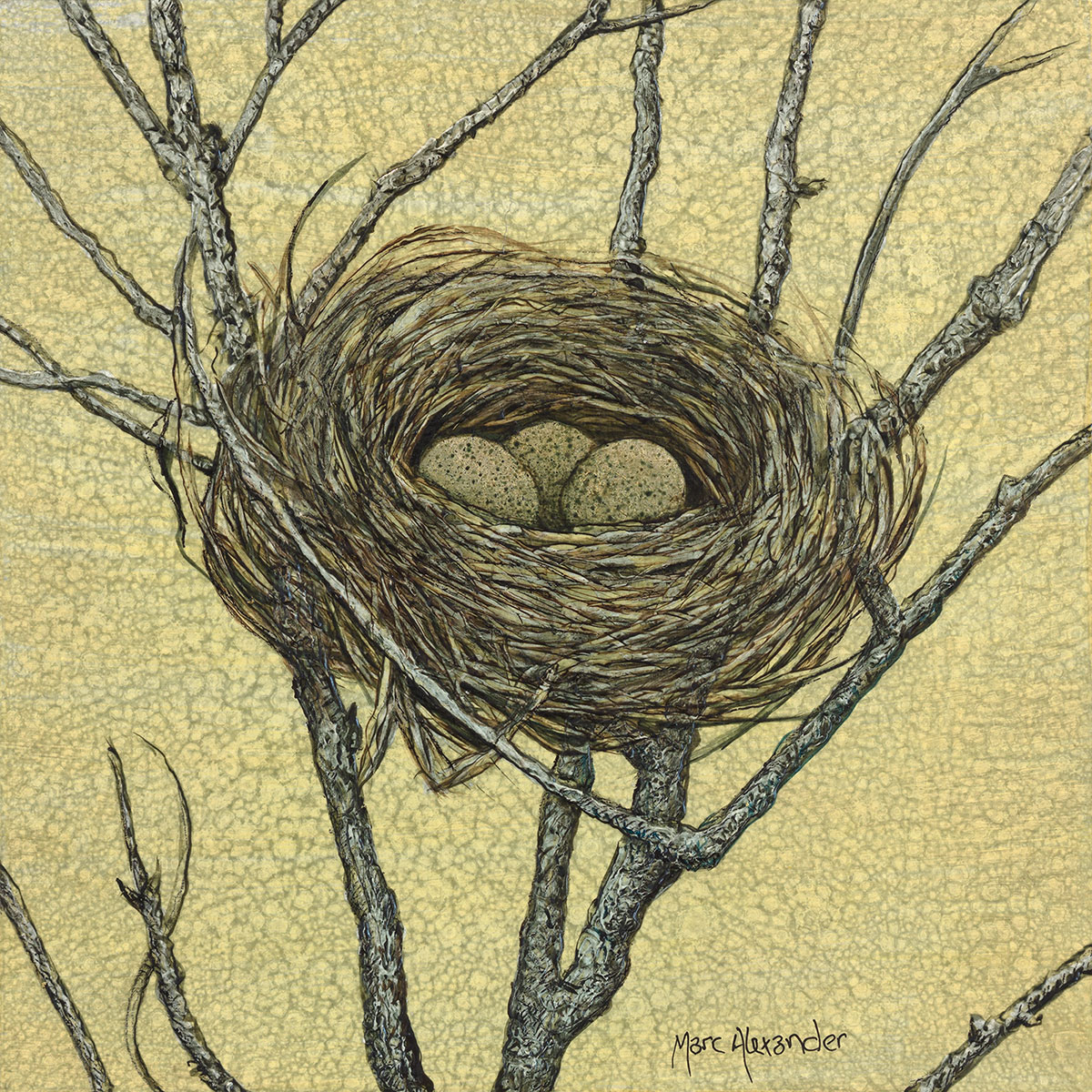##### Birds Nest I
Currency conversion from USD to: [convert number=270.00 from="usd" to="zar"] ZAR | [convert number=270.00 from="usd" to="gbp"] GBP | [convert number=270.00 from="usd" to="eur"] EUR     Medium: Oil and Gold Leaf on Board Size: 17cm by 17cm SOLD
\$ 270.00##### Birds Nest II
Currency conversion from USD to: [convert number=270.00 from="usd" to="zar"] ZAR | [convert number=270.00 from="usd" to="gbp"] GBP | [convert number=270.00 from="usd" to="eur"] EUR     Medium: Oil and Silver Leaf on Board Size: 17cm by 17cm SOLD
\$ 270.00##### Secret Path
Currency conversion from USD to: [convert number=270.00 from="usd" to="zar"] ZAR | [convert number=270.00 from="usd" to="gbp"] GBP | [convert number=270.00 from="usd" to="eur"] EUR     Medium: Oil on Board Size: 17cm by 17cm SOLD
\$ 270.00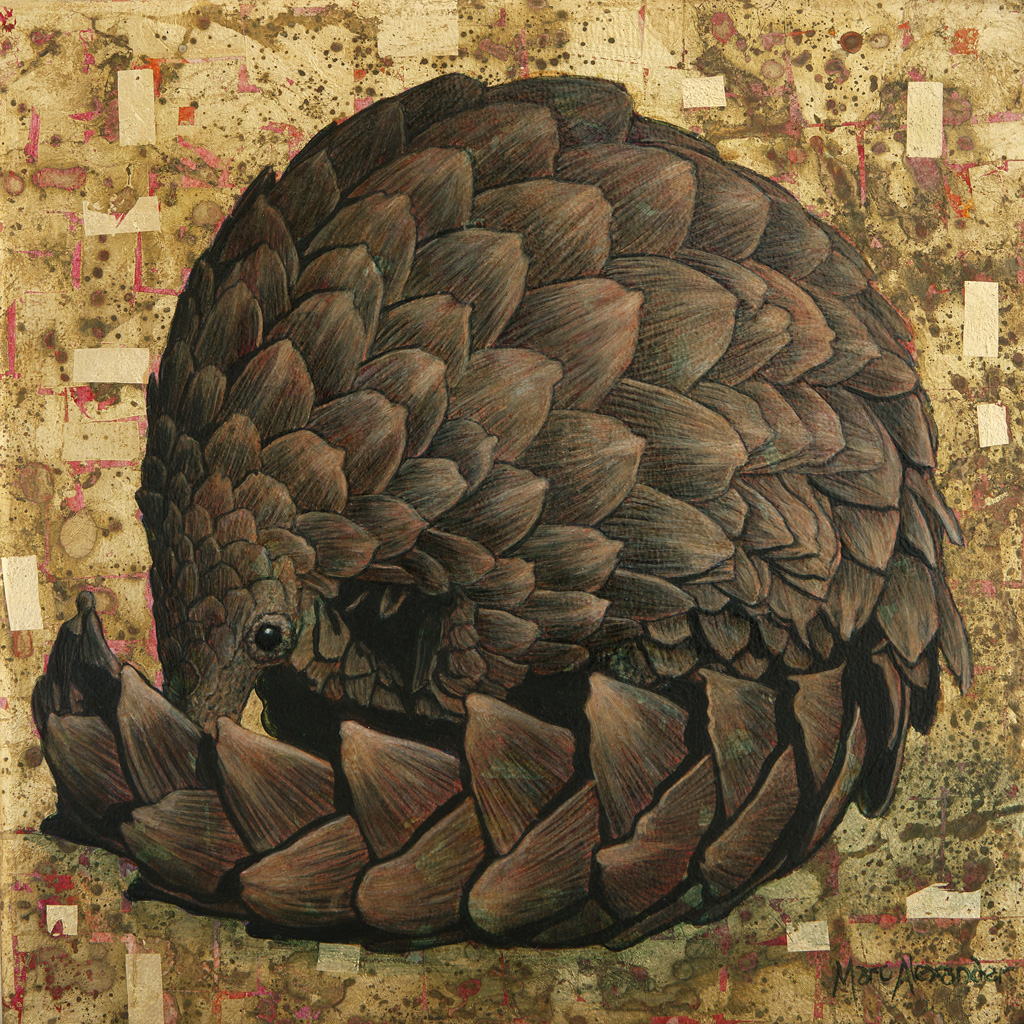##### Pangolin
Currency conversion from USD to: [convert number=650.00 from="usd" to="zar"] ZAR | [convert number=650.00 from="usd" to="gbp"] GBP | [convert number=650.00 from="usd" to="eur"] EUR     Medium: Oil and Gold Leaf on Canvas Size: 30cm by 30cm SOLD
\$ 650.00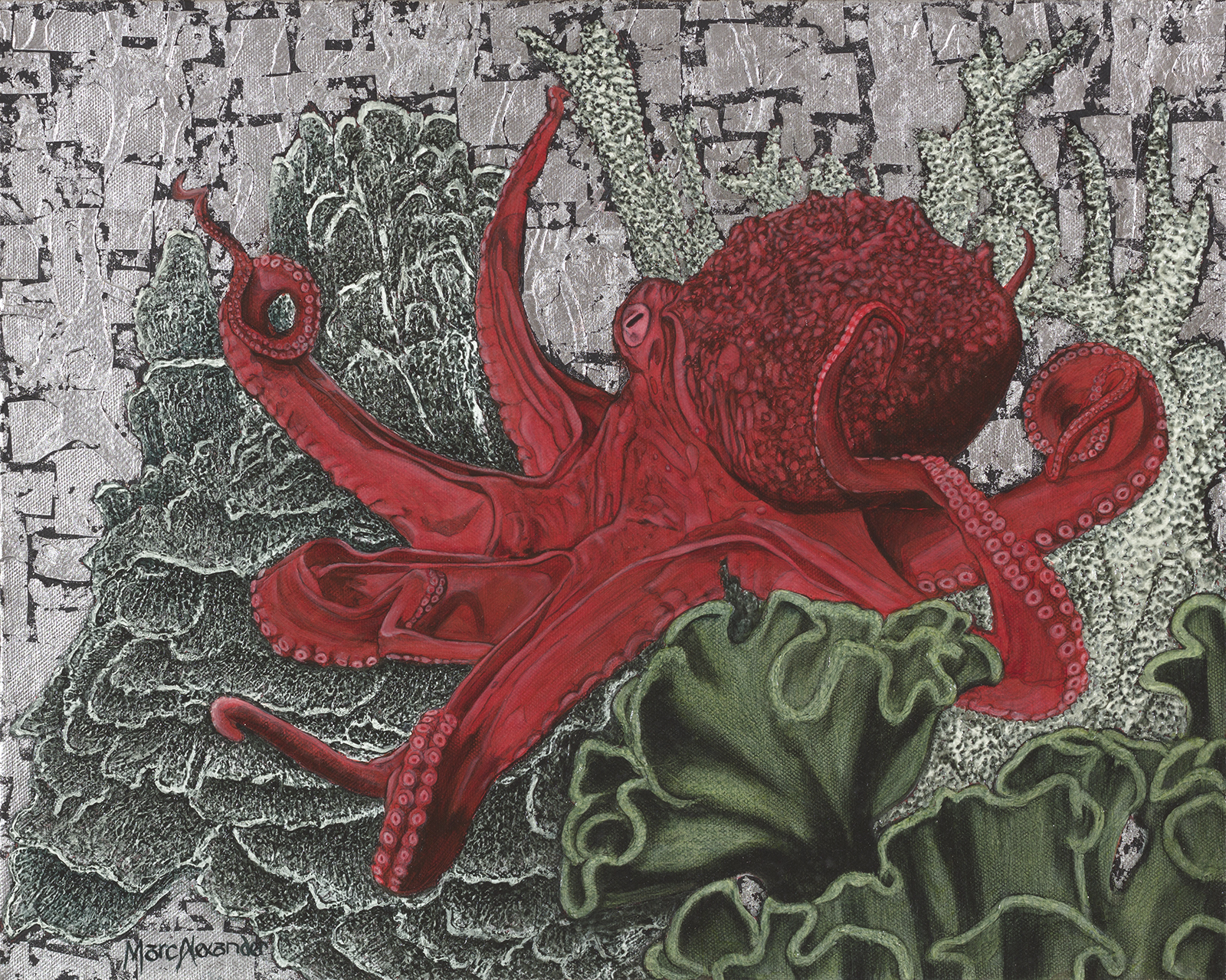##### Red Octopus
Currency conversion from USD to: [convert number=650.00 from="usd" to="zar"] ZAR | [convert number=650.00 from="usd" to="gbp"] GBP | [convert number=650.00 from="usd" to="eur"] EUR     Medium: Oil and Silver Leaf on Canvas Size: 40cm by 50cm SOLD
\$ 650.00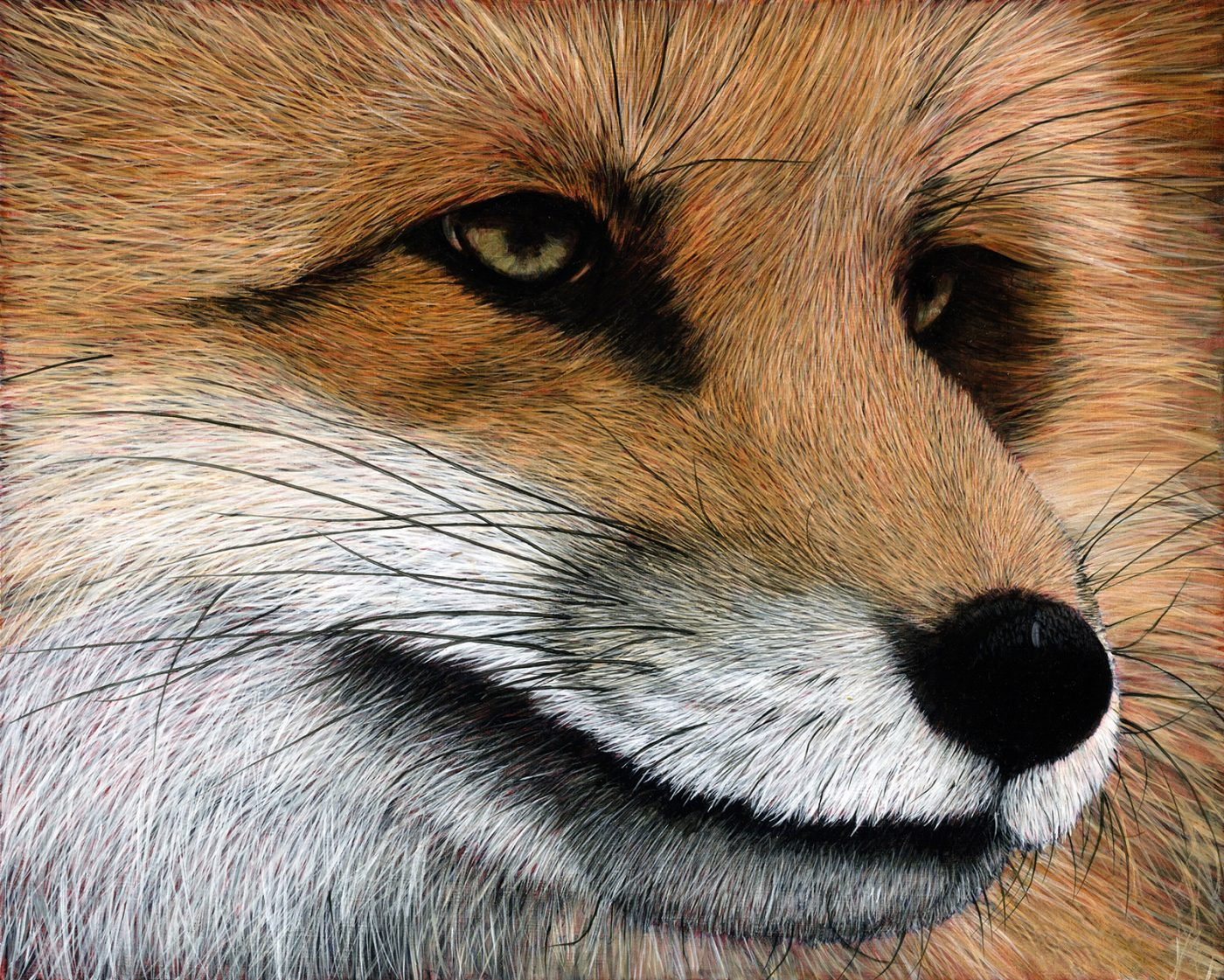##### Red Fox
Currency conversion from USD to: [convert number=650.00 from="usd" to="zar"] ZAR | [convert number=650.00 from="usd" to="gbp"] GBP | [convert number=650.00 from="usd" to="eur"] EUR     Medium: Oil on Canvas Size: 40cm by 50cm SOLD
\$ 650.00
Sale!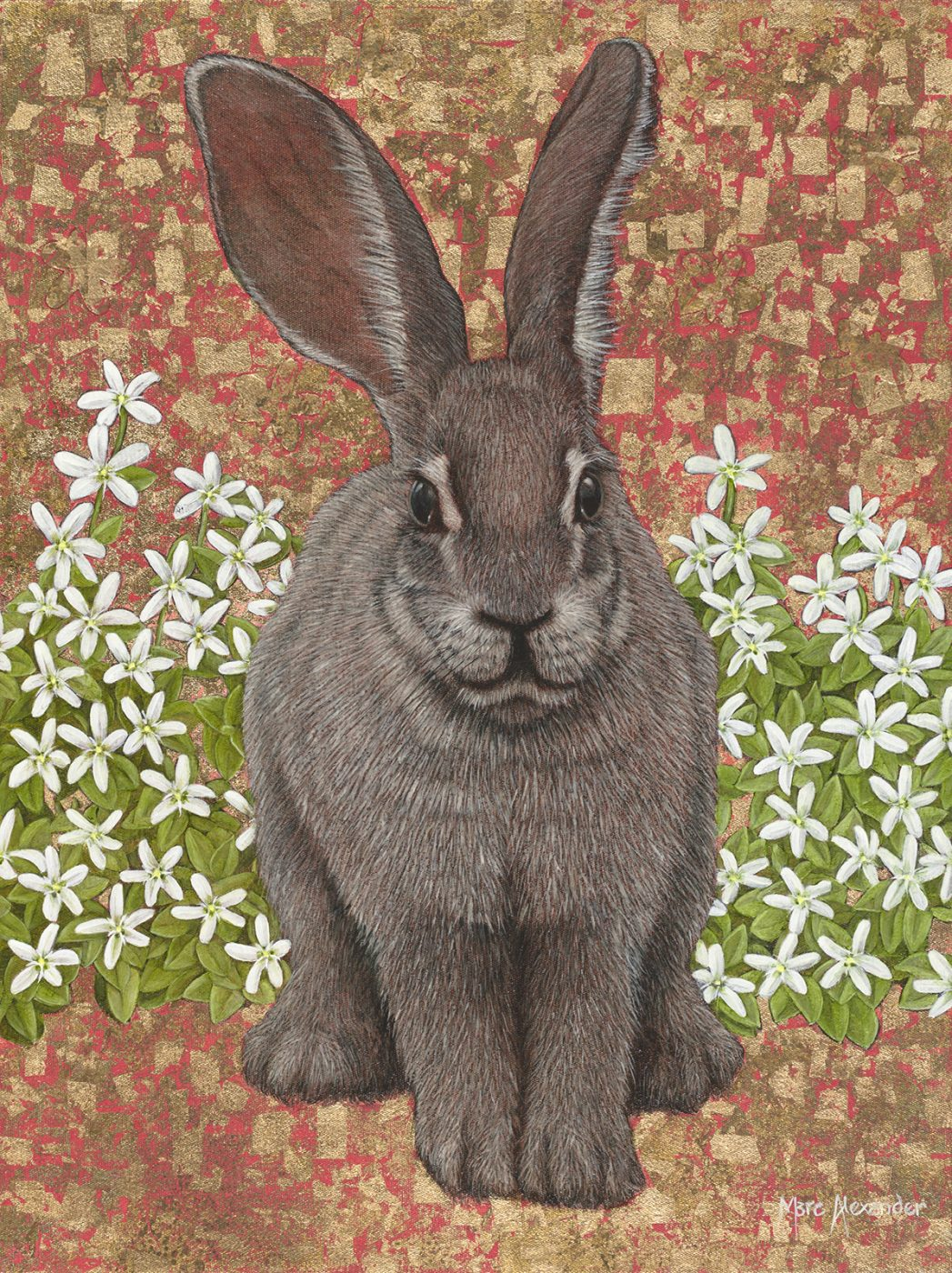##### Riverine Rabbit
Currency conversion from USD to: [convert number=700.00 from="usd" to="zar"] ZAR | [convert number=700.00 from="usd" to="gbp"] GBP | [convert number=700.00 from="usd" to="eur"] EUR     Medium: Oil and Gold Leaf on Canvas Size: 50cm by 40cm
\$ 1,350.00 \$ 700.00##### James and the Giant Tarantula
Currency conversion from USD to: [convert number=1350.00 from="usd" to="zar"] ZAR | [convert number=1350.00 from="usd" to="gbp"] GBP | [convert number=1350.00 from="usd" to="eur"] EUR     Medium: Oil and Gold Leaf on Canvas Size: 50cm by 40cm SOLD
\$ 1,350.00##### Ruby Forest
Currency conversion from USD to: [convert number=1350.00 from="usd" to="zar"] ZAR | [convert number=1350.00 from="usd" to="gbp"] GBP | [convert number=1350.00 from="usd" to="eur"] EUR     Medium: Oil and Gold Leaf on Canvas Size: 40cm by 540cm SOLD
\$ 1,350.00##### Winter Tree
Currency conversion from USD to: [convert number=1350.00 from="usd" to="zar"] ZAR | [convert number=1350.00 from="usd" to="gbp"] GBP | [convert number=1350.00 from="usd" to="eur"] EUR     Medium: Oil and Gold Leaf on Canvas Size: 40cm by 540cm SOLD
\$ 1,350.00##### Snowy Owl
Currency conversion from USD to: [convert number=1400.00 from="usd" to="zar"] ZAR | [convert number=1400.00 from="usd" to="gbp"] GBP | [convert number=1400.00 from="usd" to="eur"] EUR     Medium: Oil and Gold Leaf on Canvas Size: 74cm by 50cm SOLD
\$ 1,400.00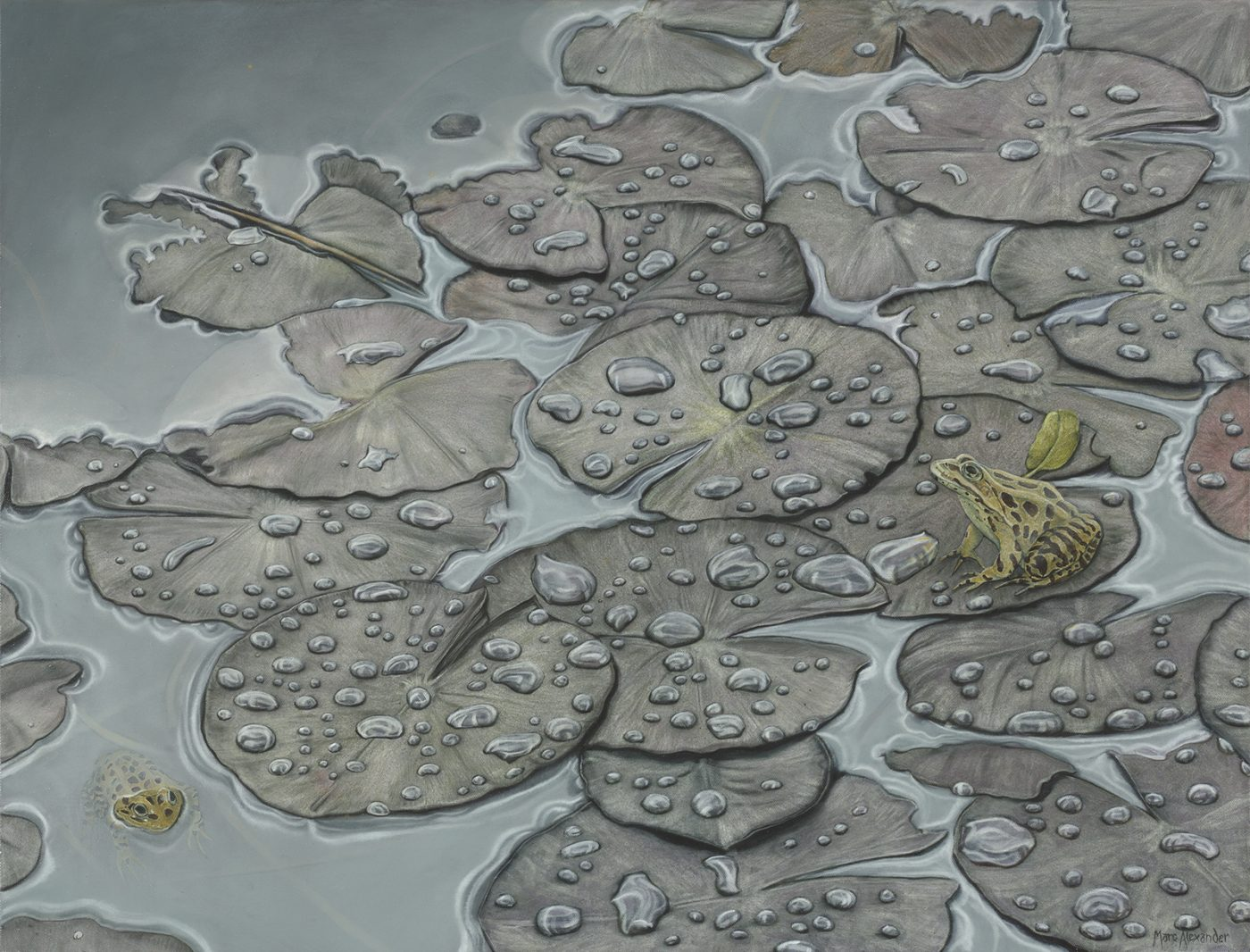Currency conversion from USD to: [convert number=1490.00 from="usd" to="zar"] ZAR | [convert number=1490.00 from="usd" to="gbp"] GBP | [convert number=1490.00 from="usd" to="eur"] EUR   Medium: Oil on Canvas Size: 68cm by 88cm SOLD
\$ 1,490.00
Sale!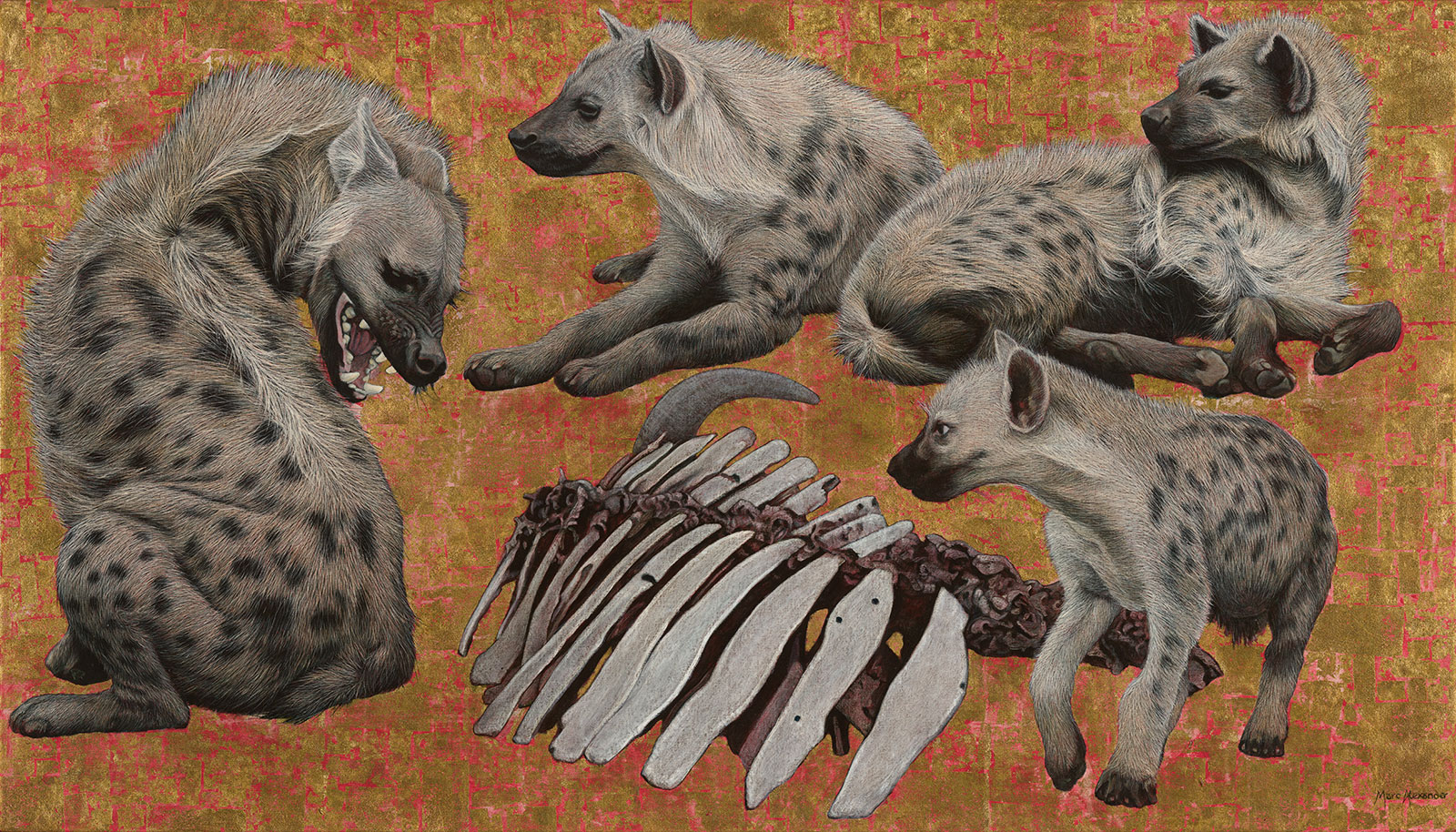##### Spotted Hyenas
Currency conversion from USD to: [convert number=1900.00 from="usd" to="zar"] ZAR | [convert number=1900.00 from="usd" to="gbp"] GBP | [convert number=1900.00 from="usd" to="eur"] EUR     Medium: Oil and Gold Leaf on Canvas Size: 80cm by 140cm
\$ 3,650.00 \$ 1,900.00
Sale!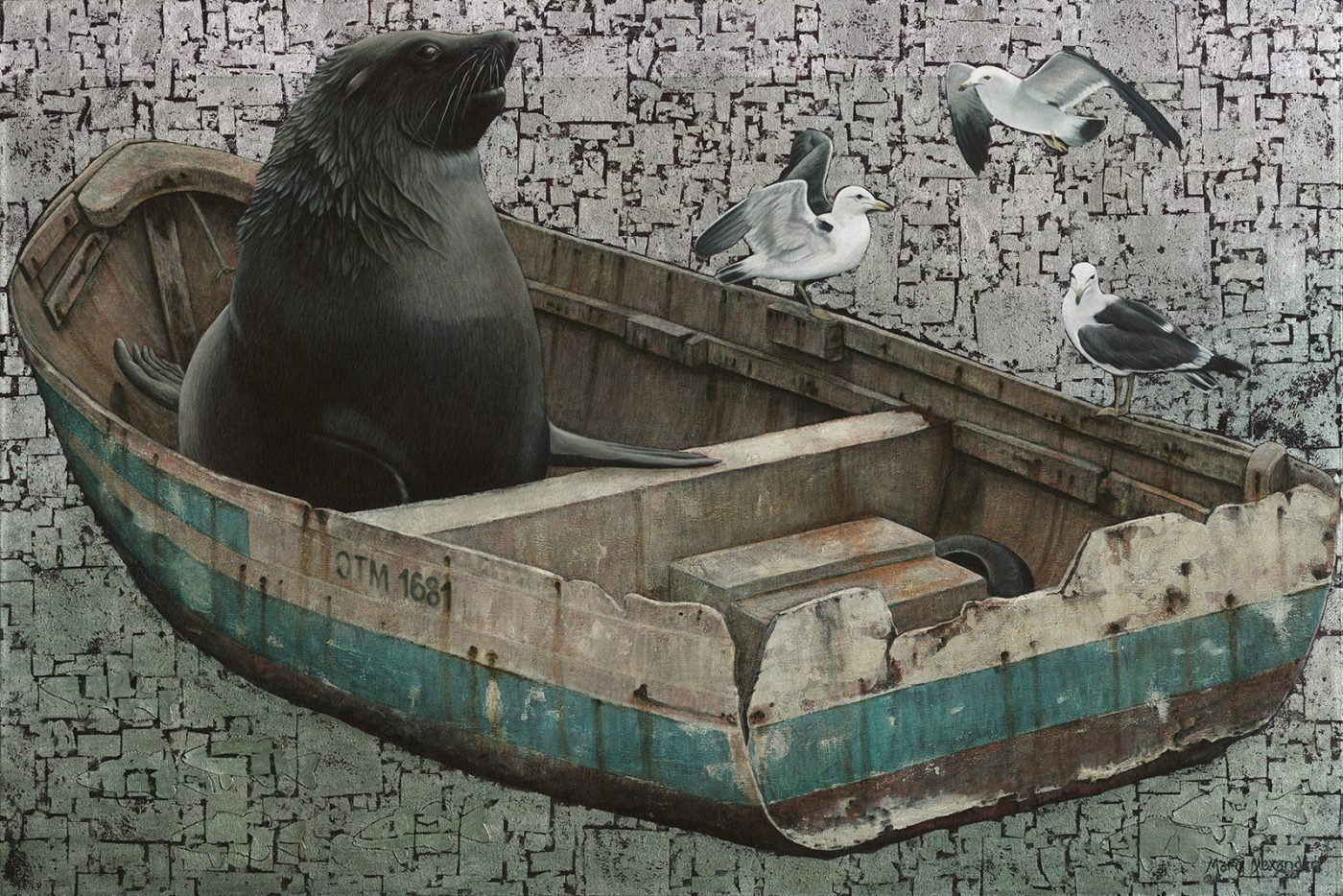##### Life of Arcto
Currency conversion from USD to: [convert number=2000.00 from="usd" to="zar"] ZAR | [convert number=2000.00 from="usd" to="gbp"] GBP | [convert number=2000.00 from="usd" to="eur"] EUR   Medium: Oil and Silver Leaf on Canvas Size: 100cm by 150cm
\$ 4,000.00 \$ 2,000.00##### Aloe I
Currency conversion from USD to: [convert number=2200.00 from="usd" to="zar"] ZAR | [convert number=2200.00 from="usd" to="gbp"] GBP | [convert number=2200.00 from="usd" to="eur"] EUR     Medium: Oil on Canvas Size: 80cm by 140cm SOLD
\$ 2,200.00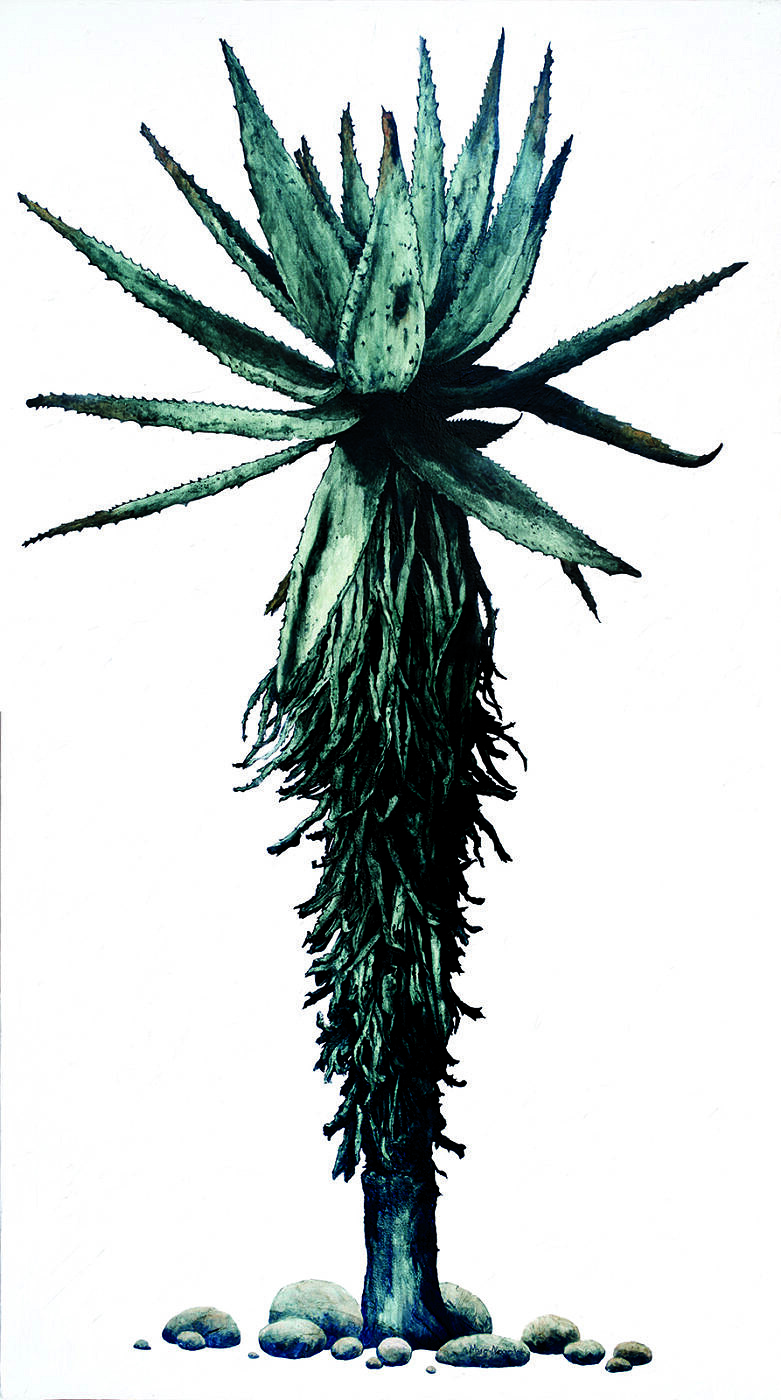##### Aloe III
Currency conversion from USD to: [convert number=2200.00 from="usd" to="zar"] ZAR | [convert number=2200.00 from="usd" to="gbp"] GBP | [convert number=2200.00 from="usd" to="eur"] EUR     Medium: Oil on Canvas Size: 80cm by 140cm SOLD
\$ 2,200.00
Sale!##### Enchanted Forest III
Currency conversion from USD to: [convert number=2200.00 from="usd" to="zar"] ZAR | [convert number=2200.00 from="usd" to="gbp"] GBP | [convert number=2200.00 from="usd" to="eur"] EUR     Medium: Oil and Gold Leaf on Canvas Size: 90cm by 120cm
\$ 3,950.00 \$ 2,200.00##### Himba Children
Currency conversion from USD to: [convert number=2200.00 from="usd" to="zar"] ZAR | [convert number=2200.00 from="usd" to="gbp"] GBP | [convert number=2200.00 from="usd" to="eur"] EUR     Medium: Oil and Gold Leaf on Canvas Size: 90cm by 120cm SOLD
\$ 2,200.00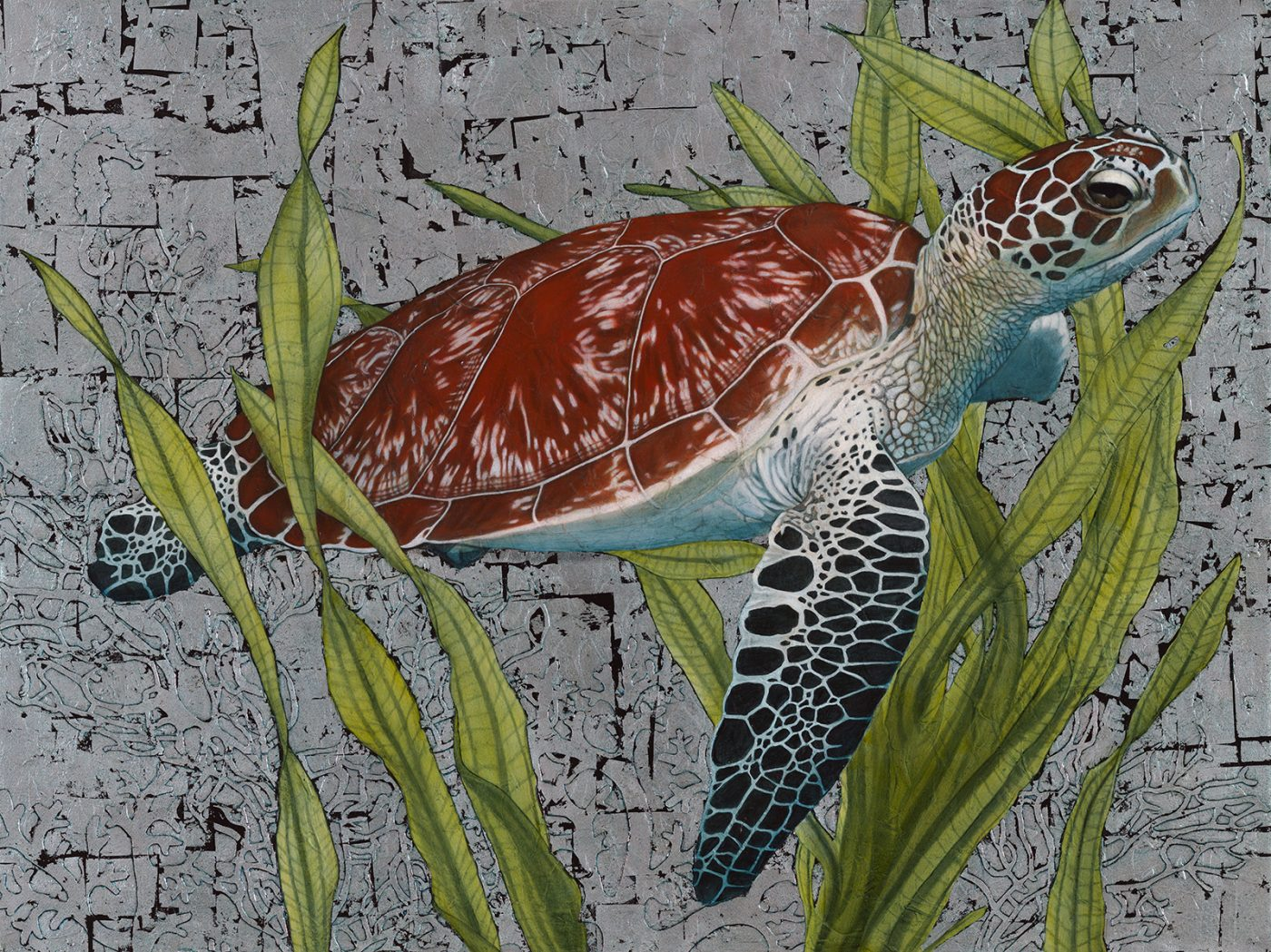##### Hawksbill Sea Turtle
Currency conversion from USD to: [convert number=2550.00 from="usd" to="zar"] ZAR | [convert number=2550.00 from="usd" to="gbp"] GBP | [convert number=2550.00 from="usd" to="eur"] EUR   Medium: Oil and Silver Leaf on Canvas Size: 90cm by 120cm SOLD
\$ 2,550.00##### Bateleur Eagle I
Currency conversion from USD to: [convert number=3450.00 from="usd" to="zar"] ZAR | [convert number=3450.00 from="usd" to="gbp"] GBP | [convert number=3450.00 from="usd" to="eur"] EUR     Medium: Oil and Gold Leaf on Canvas Size: 90cm by 120cm SOLD
\$ 3,450.00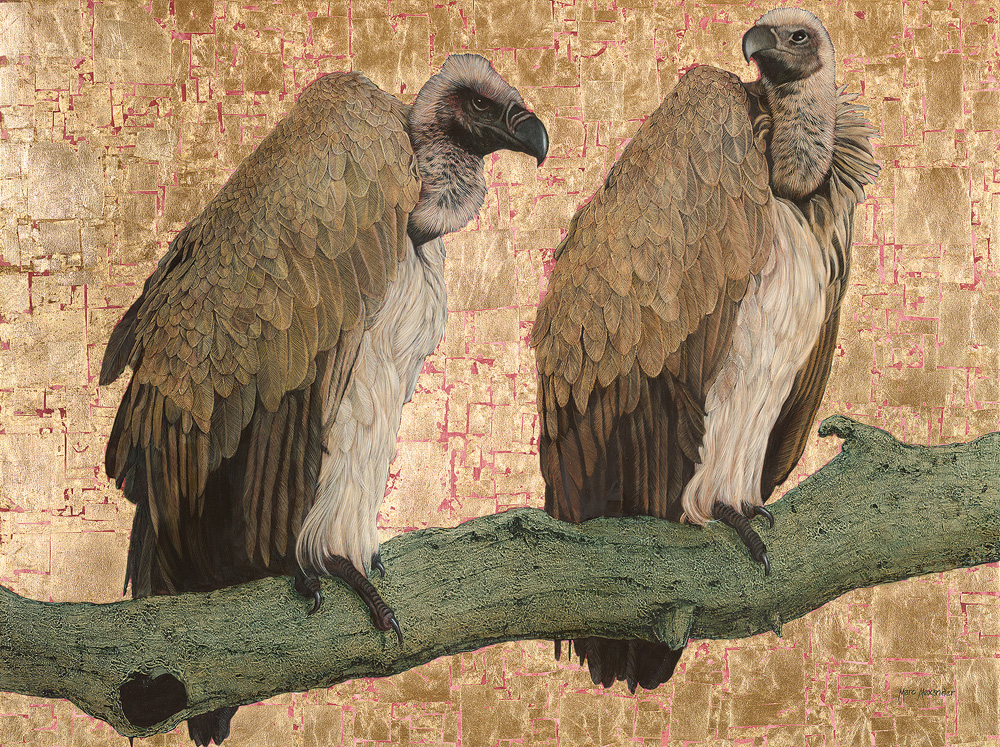##### Cape Vultures
Currency conversion from USD to: [convert number=3650.00 from="usd" to="zar"] ZAR | [convert number=3650.00 from="usd" to="gbp"] GBP | [convert number=3650.00 from="usd" to="eur"] EUR     Medium: Oil and Gold Leaf on Canvas Size: 90cm by 120cm SOLD
\$ 3,650.00##### Rhino II
Currency conversion from USD to: [convert number=3650.00 from="usd" to="zar"] ZAR | [convert number=3650.00 from="usd" to="gbp"] GBP | [convert number=3650.00 from="usd" to="eur"] EUR     Medium: Oil and Gold Leaf on Canvas Size: 90cm by 120cm SOLD
\$ 3,650.00##### Mighty Tusker
Currency conversion from USD to: [convert number=3650.00 from="usd" to="zar"] ZAR | [convert number=3650.00 from="usd" to="gbp"] GBP | [convert number=3650.00 from="usd" to="eur"] EUR     Medium: Oil and Gold Leaf on Canvas Size: 90cm by 120cm SOLD
\$ 3,650.00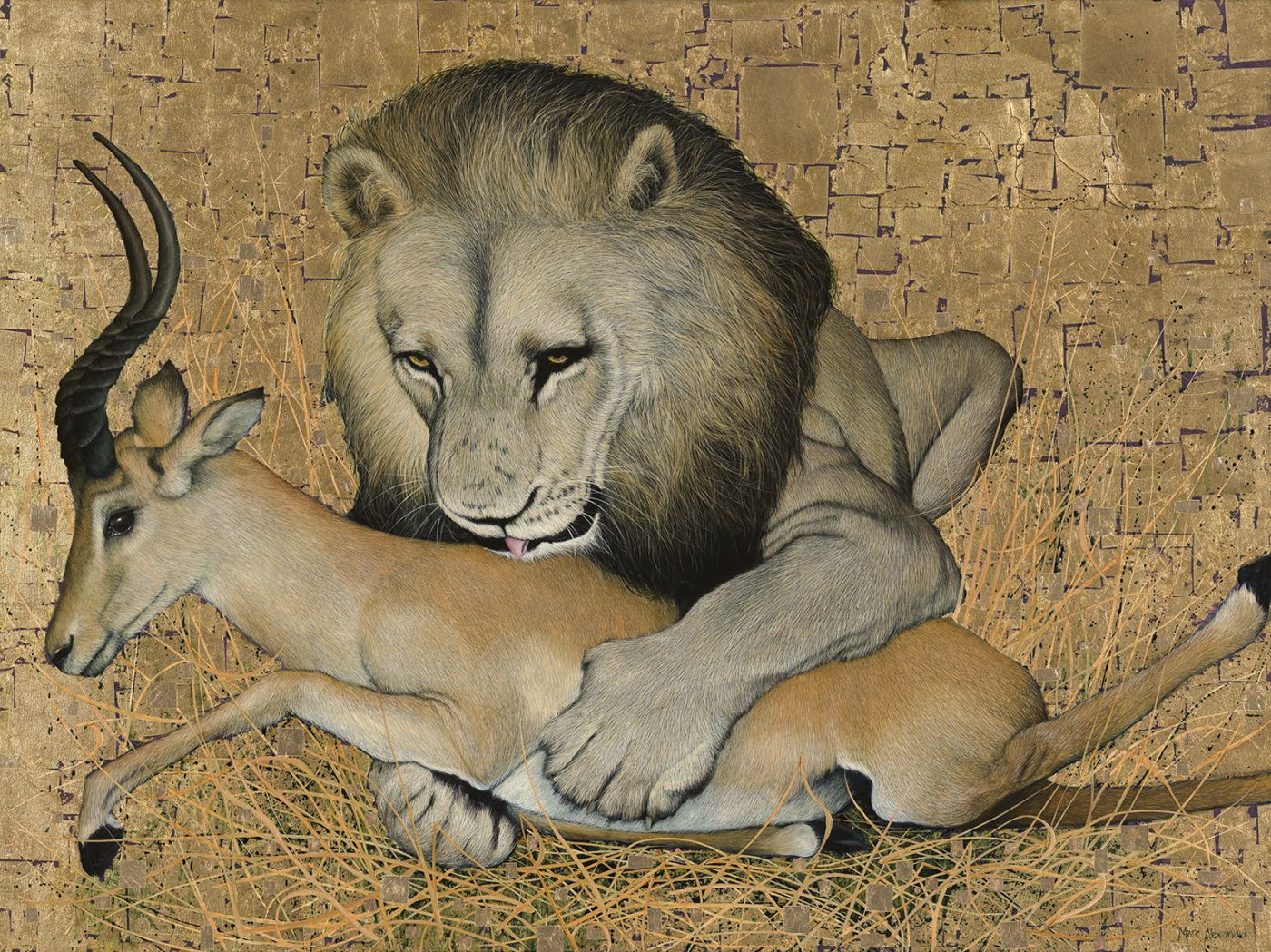##### The Kill
Currency conversion from USD to: [convert number=3650.00 from="usd" to="zar"] ZAR | [convert number=3650.00 from="usd" to="gbp"] GBP | [convert number=3650.00 from="usd" to="eur"] EUR     Medium: Oil and Gold Leaf on Canvas Size: 90cm by 120cm SOLD
\$ 3,650.00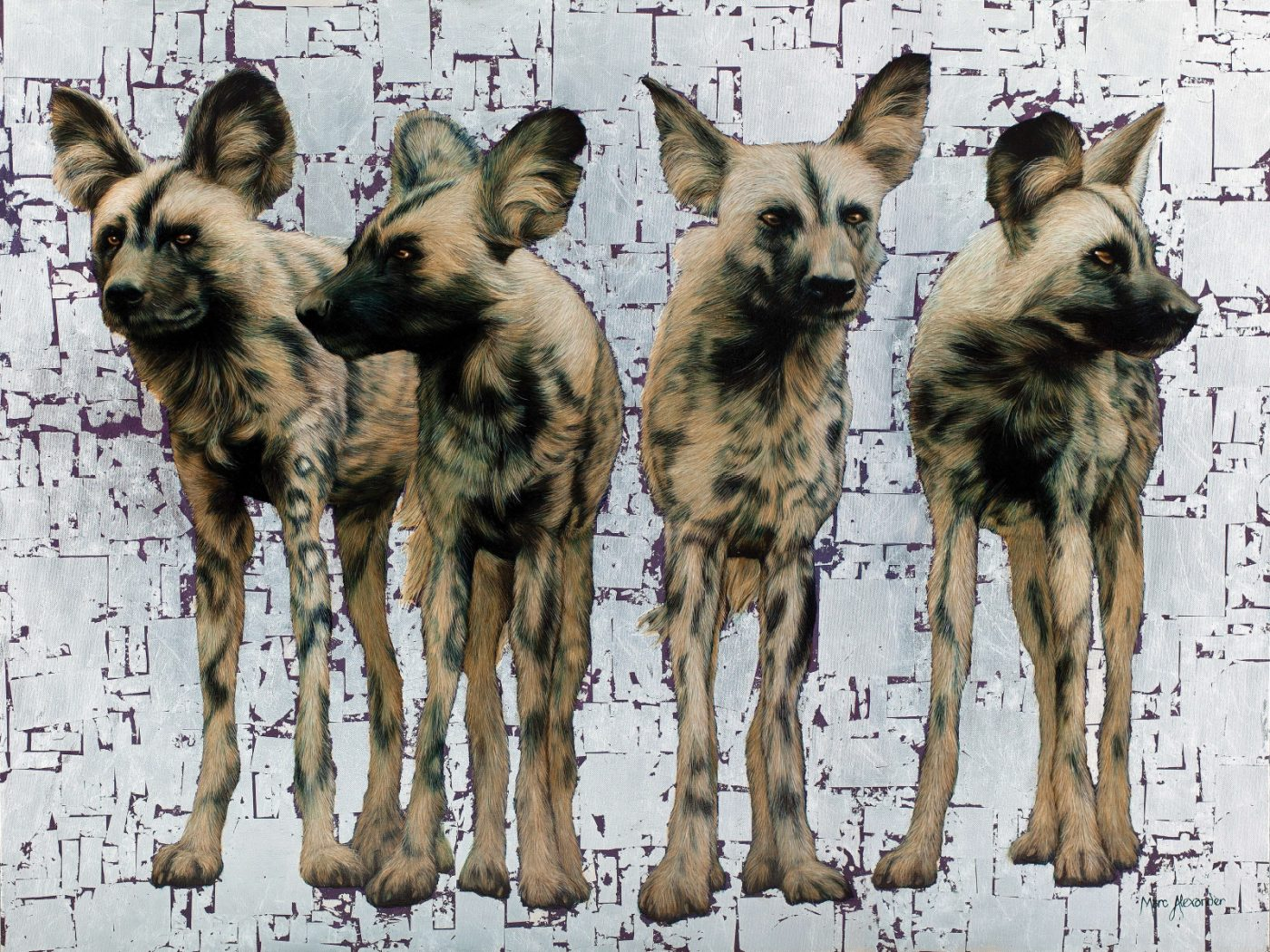##### Wild Dogs Pack of 4
Currency conversion from USD to: [convert number=3650.00 from="usd" to="zar"] ZAR | [convert number=3650.00 from="usd" to="gbp"] GBP | [convert number=3650.00 from="usd" to="eur"] EUR     Medium: Oil and Silver Leaf on Canvas Size: 90cm by 120cm SOLD
\$ 3,650.00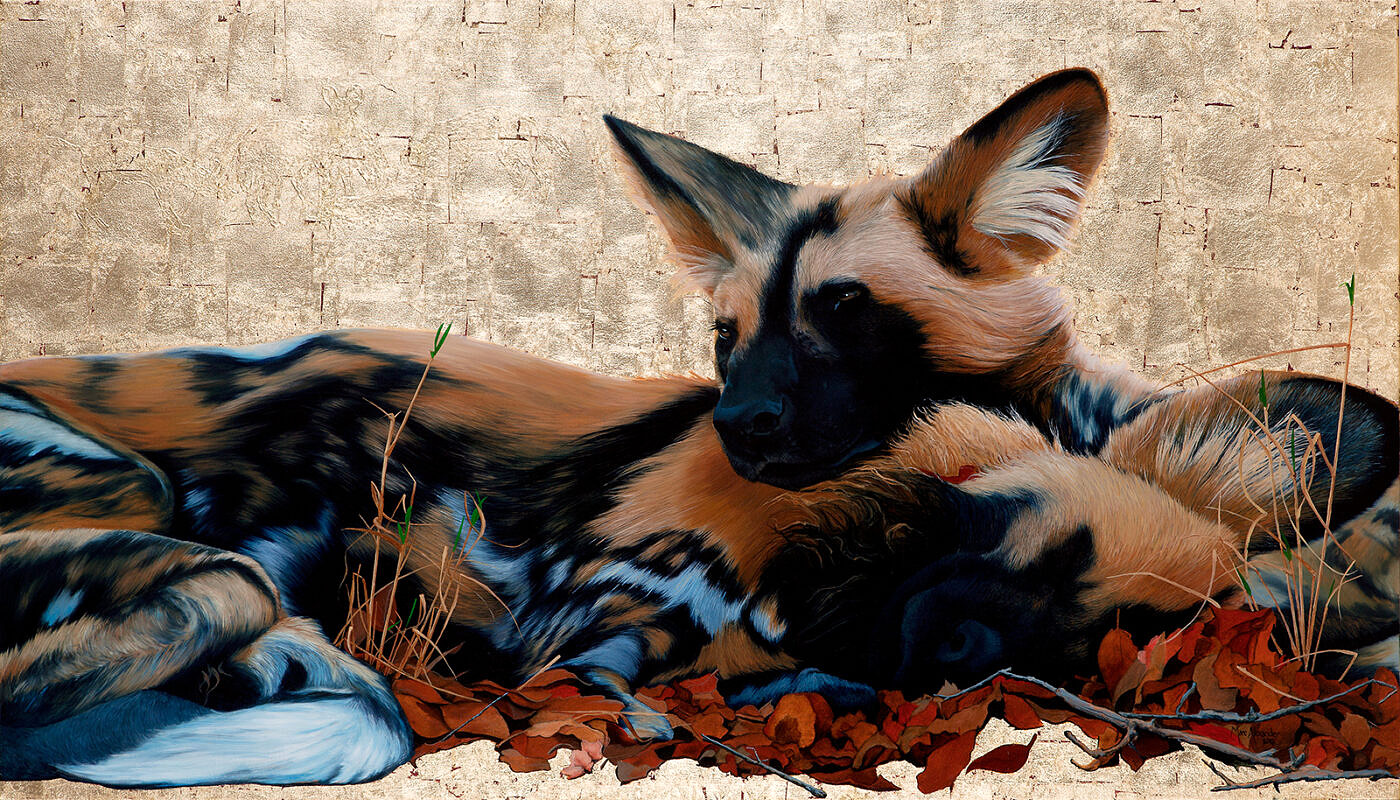##### Wild Dogs I
Currency conversion from USD to: [convert number=3650.00 from="usd" to="zar"] ZAR | [convert number=3650.00 from="usd" to="gbp"] GBP | [convert number=3650.00 from="usd" to="eur"] EUR     Medium: Oil and Gold Leaf on Canvas Size: 80cm by 140cm SOLD
\$ 3,650.00##### Wild Dogs at Dawn
Currency conversion from USD to: [convert number=3650.00 from="usd" to="zar"] ZAR | [convert number=3650.00 from="usd" to="gbp"] GBP | [convert number=3650.00 from="usd" to="eur"] EUR     Medium: Oil and Gold Leaf on Canvas Size: 80cm by 140cm SOLD
\$ 3,650.00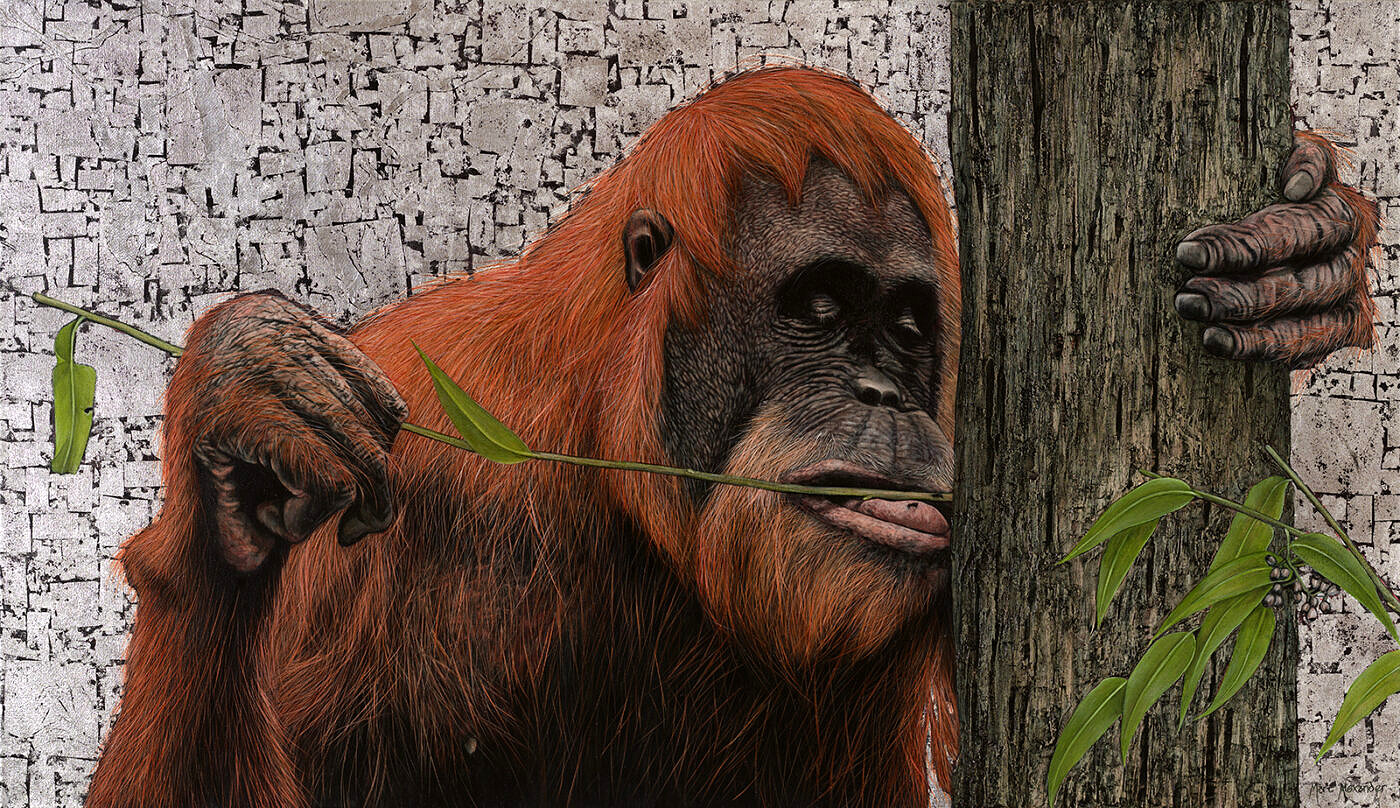##### Sumatran Orangutan
Currency conversion from USD to: [convert number=3650.00 from="usd" to="zar"] ZAR | [convert number=3650.00 from="usd" to="gbp"] GBP | [convert number=3650.00 from="usd" to="eur"] EUR     Medium: Oil and Silver Leaf on Canvas Size: 80cm by 140cm SOLD
\$ 3,650.00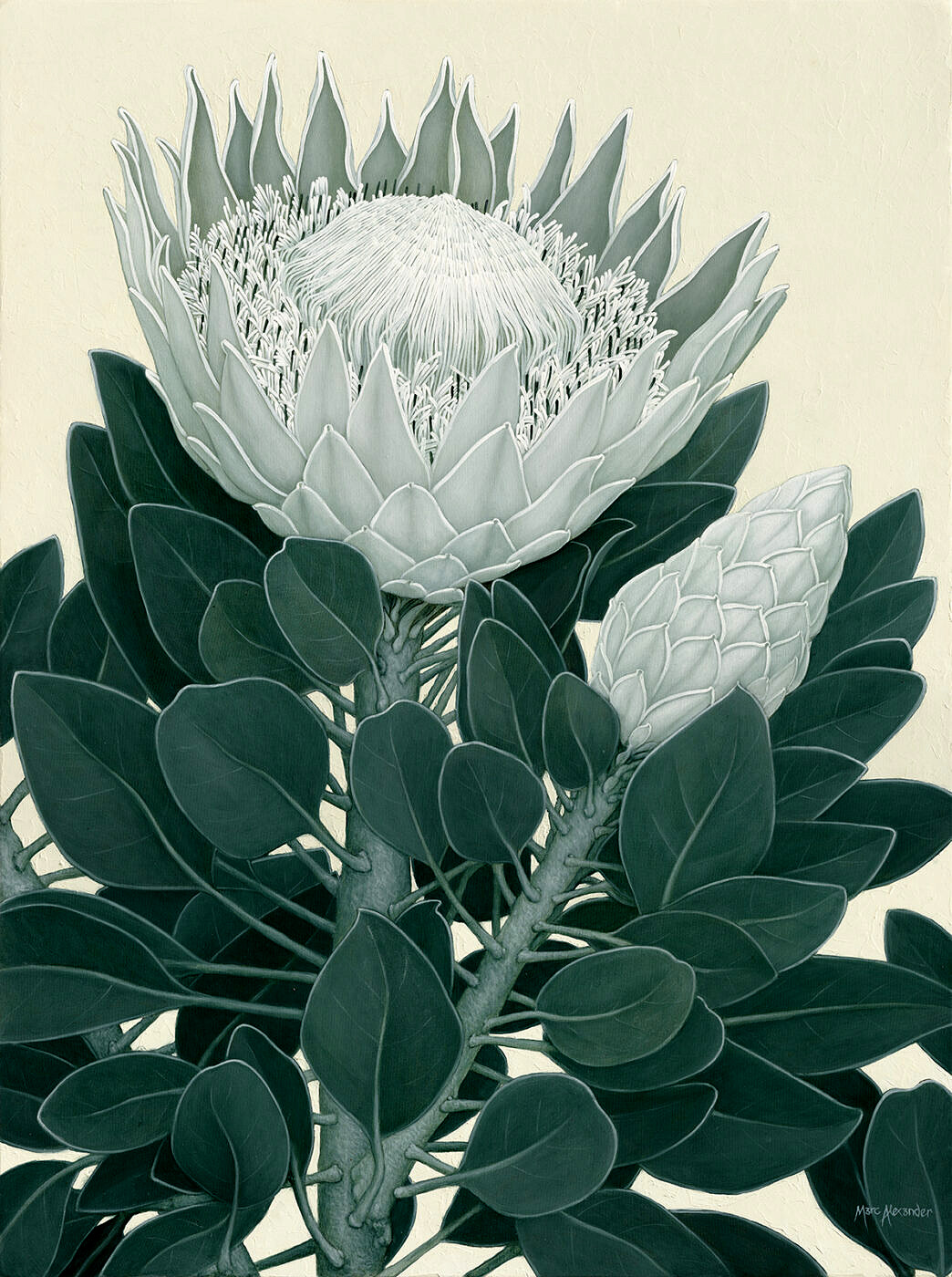##### King Protea II
Currency conversion from USD to: [convert number=3650.00 from="usd" to="zar"] ZAR | [convert number=3650.00 from="usd" to="gbp"] GBP | [convert number=3650.00 from="usd" to="eur"] EUR     Medium: Oil on Canvas Size: 1200cm by 90cm SOLD
\$ 3,650.00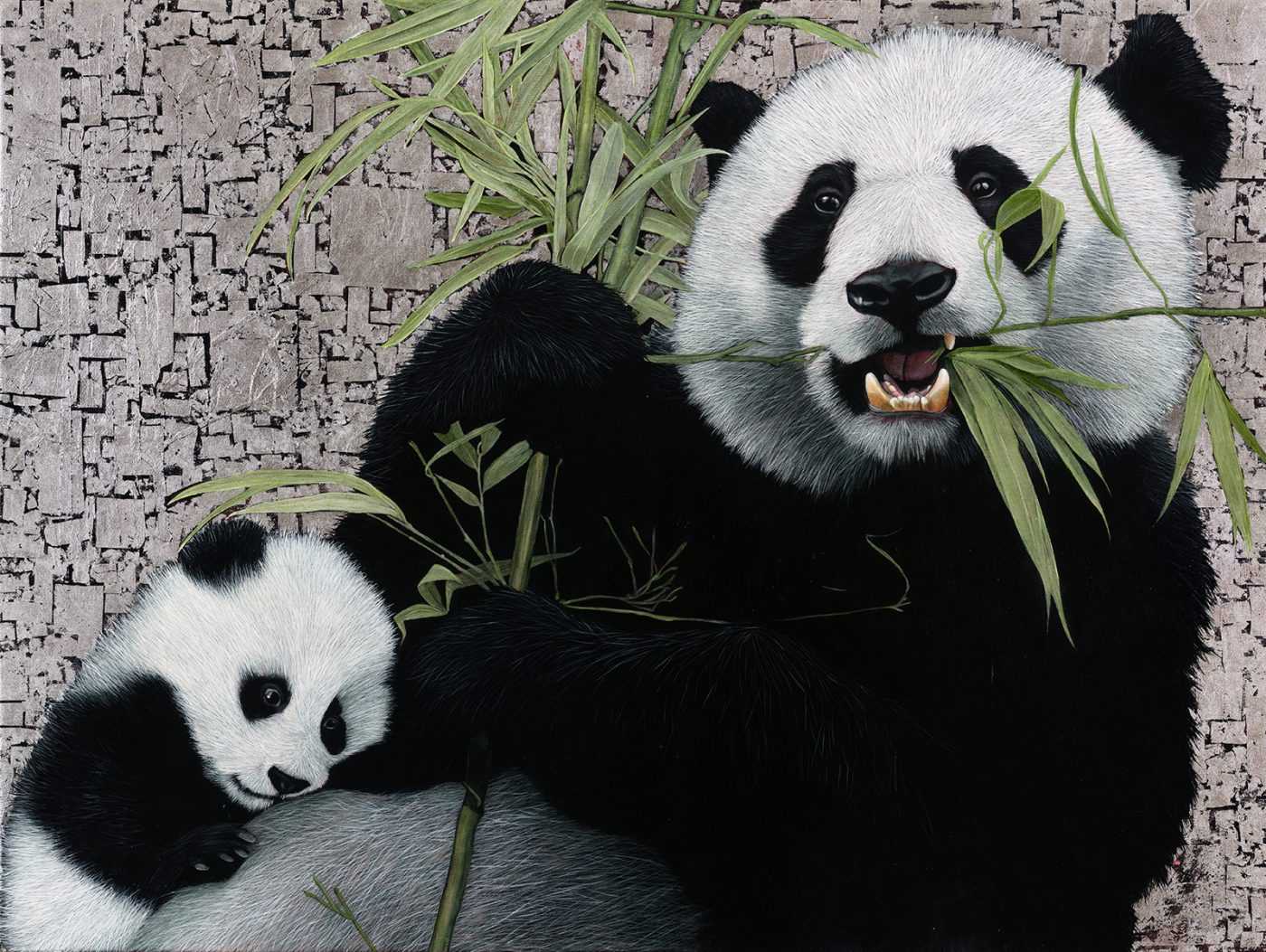##### Giant Pandas
Currency conversion from USD to: [convert number=3700.00 from="usd" to="zar"] ZAR | [convert number=3700.00 from="usd" to="gbp"] GBP | [convert number=3700.00 from="usd" to="eur"] EUR   Medium: Oil and Silver Leaf on Canvas Size: 90cm by 120cm SOLD
\$ 3,700.00##### Bateleur Eagle II
Currency conversion from USD to: [convert number=3850.00 from="usd" to="zar"] ZAR | [convert number=3850.00 from="usd" to="gbp"] GBP | [convert number=3850.00 from="usd" to="eur"] EUR     Medium: Oil and Gold Leaf on Canvas Size: 120cm by 90cm SOLD
\$ 3,850.00##### African Buffalo
Currency conversion from USD to: [convert number=3450.00 from="usd" to="zar"] ZAR | [convert number=3450.00 from="usd" to="gbp"] GBP | [convert number=3450.00 from="usd" to="eur"] EUR     Medium: Oil and Gold Leaf on Canvas Size: 90cm by 120cm SOLD
\$ 3,975.00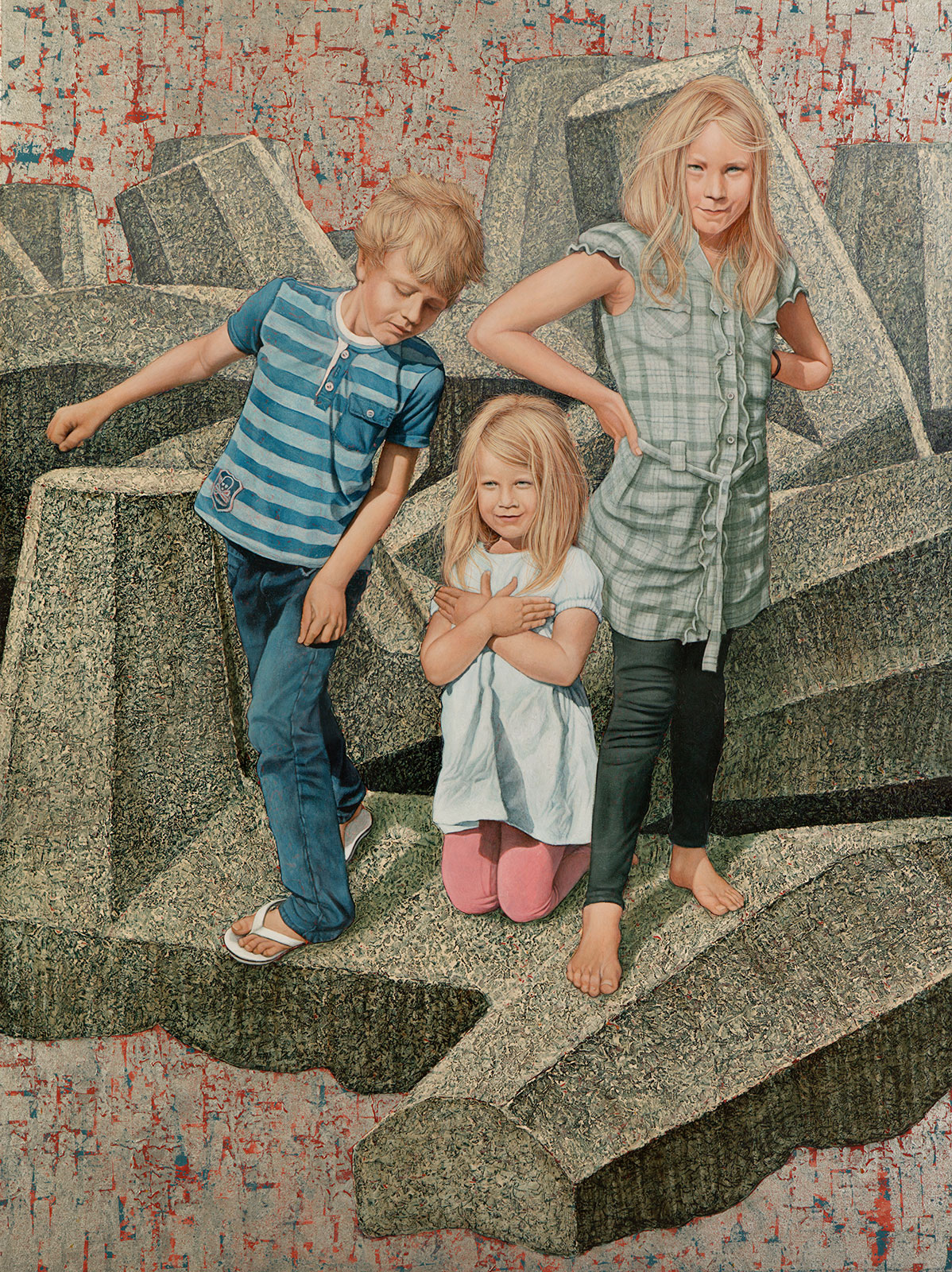##### Child’s Play
Currency conversion from USD to: [convert number=4500.00 from="usd" to="zar"] ZAR | [convert number=4500.00 from="usd" to="gbp"] GBP | [convert number=4500.00 from="usd" to="eur"] EUR     Medium: Oil and Silver Leaf on Canvas Size: 120cm by 90cm
\$ 4,500.00